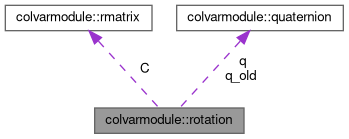Collective Variables Module - Developer Documentation
Searching...
No Matches
colvarmodule::rotation Class Reference

A rotation between two sets of coordinates (for the moment a wrapper for colvarmodule::quaternion) More...

`#include <colvartypes.h>`

Collaboration diagram for colvarmodule::rotation:[legend]

## Public Member Functions

Allocate space for the derivatives of the rotation.

Allocate space for the derivatives of the rotation.

void calc_optimal_rotation (std::vector< atom_pos > const &pos1, std::vector< atom_pos > const &pos2)
Calculate the optimal rotation and store the corresponding eigenvalue and eigenvector in the arguments l0 and q0; if the gradients have been previously requested, calculate them as well. More...

int init ()
Initialize member data.

rotation ()
Default constructor.

rotation (cvm::quaternion const &qi)
Constructor after a quaternion.

rotation (cvm::real angle, cvm::rvector const &axis)
Constructor after an axis of rotation and an angle (in radians)

~rotation ()
Destructor.

cvm::rvector rotate (cvm::rvector const &v) const
Return the rotated vector.

cvm::rotation inverse () const
Return the inverse of this rotation.

cvm::rmatrix matrix () const
Return the associated 3x3 matrix.

cvm::real spin_angle (cvm::rvector const &axis) const
Return the spin angle (in degrees) with respect to the provided axis (which MUST be normalized)

cvm::quaternion dspin_angle_dq (cvm::rvector const &axis) const
Return the derivative of the spin angle with respect to the quaternion.

cvm::real cos_theta (cvm::rvector const &axis) const
Return the projection of the orientation vector onto a predefined axis.

cvm::quaternion dcos_theta_dq (cvm::rvector const &axis) const
Return the derivative of the tilt wrt the quaternion.

## Public Attributes

cvm::quaternion q
The rotation itself (implemented as a quaternion)

cvm::real lambda
Eigenvalue corresponding to the optimal rotation.

cvm::rmatrix C
Correlation matrix C (3, 3)

cvm::matrix2d< cvm::realS
Overlap matrix S (4, 4)

cvm::vector1d< cvm::realS_eigval
Eigenvalues of S.

cvm::matrix2d< cvm::realS_eigvec
Eigenvectors of S.

cvm::matrix2d< cvm::realS_backup

std::vector< cvm::matrix2d< cvm::rvector > > dS_1
Derivatives of S.

std::vector< cvm::matrix2d< cvm::rvector > > dS_2

std::vector< cvm::rvectordL0_1

std::vector< cvm::rvectordL0_2

std::vector< cvm::vector1d< cvm::rvector > > dQ0_1

std::vector< cvm::vector1d< cvm::rvector > > dQ0_2

## Static Public Attributes

static bool monitor_crossings = false
Whether to test for eigenvalue crossing.

static cvm::real crossing_threshold = 1.0E-02
Threshold for the eigenvalue crossing test.

## Protected Member Functions

void build_correlation_matrix (std::vector< cvm::atom_pos > const &pos1, std::vector< cvm::atom_pos > const &pos2)
Build the correlation matrix C (used by calc_optimal_rotation())

void compute_overlap_matrix ()
Compute the overlap matrix S (used by calc_optimal_rotation())

## Protected Attributes

cvm::quaternion q_old
Previous value of the rotation (used to warn the user when the structure changes too much, and there may be an eigenvalue crossing)

void * jacobi
Pointer to instance of Jacobi solver.

## Detailed Description

A rotation between two sets of coordinates (for the moment a wrapper for colvarmodule::quaternion)

## ◆ calc_optimal_rotation()

 void colvarmodule::rotation::calc_optimal_rotation ( std::vector< atom_pos > const & pos1, std::vector< atom_pos > const & pos2 )

Calculate the optimal rotation and store the corresponding eigenvalue and eigenvector in the arguments l0 and q0; if the gradients have been previously requested, calculate them as well.

The method to derive the optimal rotation is defined in: Coutsias EA, Seok C, Dill KA. Using quaternions to calculate RMSD. J Comput Chem. 25(15):1849-57 (2004) DOI: 10.1002/jcc.20110 PubMed: 15376254

The documentation for this class was generated from the following files: